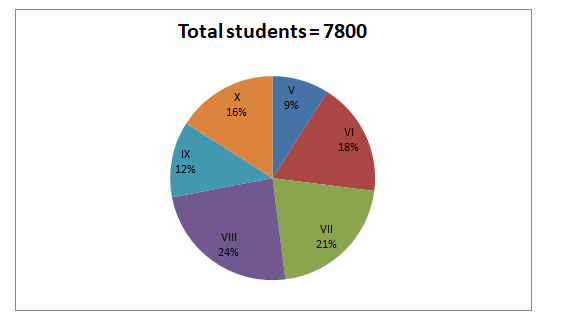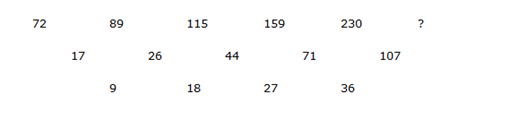# “20-20” Quantitative Aptitude | Crack SBI Clerk Prelims 2018 Day-100

Dear Readers, Find the aptitude test questions to crack latest bank exams. We regularly provide 20 aptitude test questions daily for students. Aspirants practice these questions on a regular basis to improve your score in aptitude section. Aspirants preparing for the exams can make use of this “20-20” Quantitative Aptitude Questions. SBI Clerk Prelims 2018 Notification has been released we hope you all have started your preparation. Here we have started New Series of Practice Materials specially for SBI Clerk Prelims 2018. Aspirants those who are preparing for the exams can use this “20-20” Quantitative Aptitude Questions.

“20-20” Aptitude Questions | Crack SBI Clerk 2018 Day-100

Click “Start Quiz” to attend these Questions and view Solutions

Directions (Q. 1 – 5): What value should come in place of question mark (?) in the following questions?

1. (3365 ÷ 67.3) % of 8472 +? % of 750 = 5736
1. 164
2. 175
3. 200
4. 182
5. 193
1. 210 % of 250 +? % of 575 = 600
1. 15 2/7
2. 13 1/23
3. 11 5/17
4. 9 4/27
5. 17 2/29
1. 480 % of 750 + (?) of 8 – 40 % of 5550 = 7292
1. 657
2. 821
3. 598
4. 739
5. 934
1. 1.8 × 625 + 5.6 ÷ 0.7 + 415 × 11.8 = (?)2 – 211
1. 79
2. 85
3. 92
4. 69
5. 61
1. 45 % of 480 +? % of 250 = 50 % of 748
1. 56.7
2. 48.9
3. 52.4
4. 63.2
5. 43.5

Directions (Q. 6 – 10): What value should come in place of question mark (?) in the following number series?

1. 56, 227, 913,? , 14645
1. 2346
2. 2891
3. 4563
4. 3248
5. 3659
1. 72, 89, 115, 159, 230,?
1. 337
2. 421
3. 293
4. 374
5. 468
1. 111, 112, 228, 693,?
1. 1894
2. 2346
3. 1672
4. 2788
5. 3240
1. 278, 343, 469, 686, 1030,?
1. 1784
2. 1543
3. 1367
4. 1978
5. 2236
1. 8, 21, 79, 214,? , 843
1. 532
2. 629
3. 458
4. 477
5. 594
1. The simple interest accrued on an amount of Rs. 22000 at the end of three years is Rs. 3960. Find the corresponding Compound interest (approx)?
1. 3578
2. 3956
3. 4202
4. 4634
5. 4890
1. Ravi sells an article at a gain of 15 %. Had he sold it for Rs. 3780 more, he would have gained 20 %. Find the cost price of article.
1. 75600
2. 65800
3. 70300
4. 63900
5. None of these
1. Arun started a business by investing Rs. 56000. After 5 months, Bharathi joined him with a capital of Rs. 48000. At the end of the year, Bharathi received Rs. 3250 as share of profit. What is the total profit at the end of the year?
1. 8670
2. 7830
3. 9240
4. 8260
5. 9750
1. A train whose speed is 30 m/s crosses a bridge in 15 seconds. Another train which is 70 m shorter than the first train crosses the same bridge at the speed of 54 km/hr. Find the time taken by the second train to cross the bridge.
1. 76/3 sec
2. 81/4 sec
3. 56/5 sec
4. 92/7 sec
5. None of these
1. Three years ago, the ages of P and Q was 2: 3. Seven years hence, the ages of P and Q is 4: 5. Find the sum of the present age of P and Q?
1. 27 years
2. 31 years
3. 33 years
4. 35 years
5. 29 years

Directions (Q. 16 – 20) Study the following information carefully and answer the given questions:

Following pie chart shows the total number of students studying in different standards and the table shows the ratio of boys and girls among them.Standards Boys : Girls V 5 : 4 VI 1 : 2 VII 2 : 1 VIII 4 : 5 IX 1 : 1 X 3 : 1
1. Total number of girls in the standard VI, VII and X together is approximately what percentage of total number of boys in the standard V, VIII and IX together?
1. 115 %
2. 106 %
3. 97 %
4. 88 %
5. 123 %
1. Find the ratio of total number of students in the standard V and VI together to that of total number of boys in the standard VII, IX and X together?
1. 179 : 217
2. 256 : 351
3. 324 : 479
4. 351 : 416
5. None of thes
1. Find the total number of girls of all the given standards together?
1. 2956
2. 3867
3. 3614
4. 4115
5. 2743
1. Find the difference between the total number of girls in the standard V, VII and IX together to that of total number of boys in the standard VI, VIII and X together?
1. 910
2. 825
3. 750
4. 830
5. None of these
1. Total number of students in the standard V, VI and IX together is approximately what percentage more/less than the total number of students in the standard VIII and X together?
1. 12 % less
2. 7 % less
3. 9 % more
4. 14 % more
5. 3 % less

Directions (Q. 1 – 5):

(3365/67.3) % of 8472 +? % of 750 = 5736

50 % of 8472 + (x/100)*750 = 5736

(50/100)*8472 + (15x/2) = 5736

4236 + (15x/2) = 5736

(15x/2) = 5736 – 4236

(15x/2) = 1500

X = 1500*(2/15)

X = 200

(210/100)*250 + (x/100)*575 = 600

525 + (23x/4) = 600

(23x/4) = 600 – 525

(23x/4) = 75

X = 75*(4/23)

X = 300/23 = 13 1/23

(480/100)*750 + 8x – (40/100)*5550 = 7292

3600 + 8x – 2220 = 7292

8x = 7292 – 3600 + 2220

X = 5912/8

X = 739

1.8 × 625 + 5.6 ÷ 0.7 + 415 × 11.8 = (?)2 – 211

1125 + 8 + 4897 = x2 – 211

6241 = x2

X = 79

(45/100)*480 + (x/100)*250 = (50/100)*748

216 + (5x/2) = 374

(5x/2) = 374 – 216

X = 158*(2/5)

X = 63.2

Directions (Q. 6 – 10):

The pattern is, *4 + 3, *4 + 5, *4 + 7, *4 + 9…The pattern is, *1 + 12, *2 + 22, *3 + 32, *4 + 42

The difference is, 43 + 1, 53 + 1, 63 + 1, 73 + 1, 83 + 1…

The pattern is, +(42 – 3), +(82 – 6), +(122 – 9), +(162 – 12), +(202 – 15),..

S.I = PNR/100

3960 = 22000*3*R/100

R = (3960*100)/(22000*3) = 6 %

Corresponding C.I:

22000*(6/100) = 1320

23320*(6/100) = 1399.2

24719.2*(6/100) = 1483.152

C.I = 1320 + 1399.2 + 1483.152 = Rs. 4202.152 = Rs. 4202

According to the question,

(20 % – 15 %) of cost price = 3780

5 % of cost price = 3780

Cost price = 3780*(100/5) = Rs. 75600

The share of Arun and Bharathi

= > [56000*12]: [48000*7]

= > 672000: 336000

= > 2: 1

The share of Bharathi = Rs. 3250

Total profit = 3250*3 = Rs. 9750

Distance covered by the first train= 30*15= 450m

Distance covered by the second train= 450-70= 380m

Time taken by the second train to cross the bridge

= 380/(54*5/18)= 380/3*5= (76/3) seconds

Three years ago, the ages of P and Q = 2: 3(2x, 3x)

Seven years hence, the ages of P and Q = 4: 5

According to the question,

(2x + 10)/(3x + 10) = (4/5)

10x + 50 = 12x + 40

10 = 2x

X = 5

Present ages are, P = 2x + 3 = 13

Q = 3x + 3 = 18

Sum of Present age of P and Q = 13 + 18 = 31 years

Directions (Q. 16 – 20)

 Standards Total number of students Boys Girls V 702 390 312 VI 1404 468 936 VII 1638 1092 546 VIII 1872 832 1040 IX 936 468 468 X 1248 936 312

Total number of girls in the standard VI, VII and X together

= > 936 + 546 + 312 = 1794

Total number of boys in the standard V, VIII and IX together

= > 390 + 832 + 468 = 1690

Required % = (1794/1690)*100 = 106.15 % = 106 %

Total number of students in the standard V and VI together

= > 702 + 1404 = 2106

Total number of boys in the standard VII, IX and X together

= > 1092 + 468 + 936 = 2496

Required ratio = 2106: 2496 = 351: 416

Total number of girl students of all the given standards together

= > 312 + 936 + 546 + 1040 + 468 + 312

= > 3614

The total number of girls in the standard V, VII and IX together

= > 312 + 546 + 468 = 1326

The total number of boys in the standard VI, VIII and X together

= > 468 + 832 + 936 = 2236

Required difference = 2236 – 1326 = 910

Total number of students in the standard V, VI and IX together

= > [(9 + 18 + 12)/100]*7800

= > (39/100)*7800

Total number of students in the standard VIII and X together

= > [(24 + 16)/100]*7800

= > (40/100)*7800

Required % = {[(40/100)*7800 – (39/100)*7800]/ ((40/100)*7800)}*100

= > (1/40)*100

= > 2.5 % = 3 % less

Daily Practice Test Schedule | Good Luck

 Topic Daily Publishing Time Daily News Papers & Editorials 8.00 AM Current Affairs Quiz 9.00 AM Quantitative Aptitude “20-20” 11.00 AM Vocabulary (Based on The Hindu) 12.00 PM General Awareness “20-20” 1.00 PM English Language “20-20” 2.00 PM Reasoning Puzzles & Seating 4.00 PM Daily Current Affairs Updates 5.00 PM Data Interpretation / Application Sums (Topic Wise) 6.00 PM Reasoning Ability “20-20” 7.00 PM English Language (New Pattern Questions) 8.00 PM